# Liquid level measurement using hydrostatic pressure and buoyancy

## Instrumentation and Process Control

• #### Question 1

A vessel containing a weak acid/water mixture has a calibrated level range of 0 to 10 feet, measured by a pressure transmitter at the bottom. Suppose that the specific gravity of the normal mixture is 1.12 (112

Now, suppose some pure acid is added to the vessel, increasing the actual liquid level {\it and} increasing the liquid density at the same time. The new liquid level is 7 feet high and the specific gravity has increased to 1.35. Assuming the transmitter has been calibrated for the original specific gravity of 1.12, what will its output signal correspond to in feet of liquid level? In other words, if this transmitter’s output signal were driving a level indicator device for an operator to read, how many feet of level would the indicator device register?

Identify an alternative method for liquid level measurement that would not be affected by changes in liquid density.

Reveal answer
• #### Question 2

An open vessel contains water at 60$^{o}$ F. A pressure transmitter located at the bottom of the vessel measures the hydrostatic pressure (head’‘) generated by the water and outputs a signal corresponding to level. Suppose that the temperature of this vessel were to increase over time to 110$^{o}$ F due to exposure to very hot outside air (the vessel is located in Death Valley, California during the summer). Knowing that an increase in water temperature will result in a decrease in density, what will happen to the level transmitter’s output as the vessel heats up? Will the transmitter output increase, decrease, or stay the same? Why?? Assume that no water enters or exits the vessel during the period of heating from 60$^{o}$ F to 110$^{o}$ F.

Reveal answer
• #### Question 3

A vessel holding some process liquid needs to have its level monitored. The range of level in this vessel is 0 to 20 feet, and the process liquid has a specific gravity of 1.0 (like water). Someone decides to attach a pressure transmitter to the bottom of the vessel to infer level from hydrostatic pressure like this: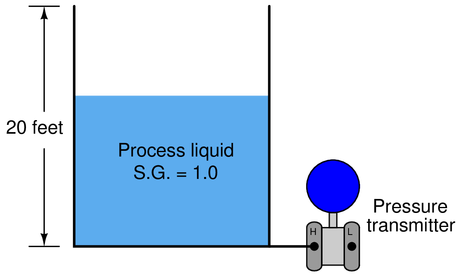Later, a top is added to this vessel to keep rain from entering in. Unfortunately, though, this process liquid tends to emit vapor which will be trapped by the closed vessel and create a pressure inside of it. A small vent is added to the top of the vessel to permit the vapor to escape, but it is a {\it small} vent, not big enough to ensure a total absence of vapor pressure buildup at all times:What problem in level measurement will result from there being an occasional vapor pressure buildup inside this vessel? How may this problem be corrected so that the liquid level will be accurately measured at all times?

Reveal answer
• #### Question 4

The principle of buoyancy may be used to create a level transmitter instrument, generating an output signal proportional to the change in weight of a displacer’’ rod suspended in a liquid: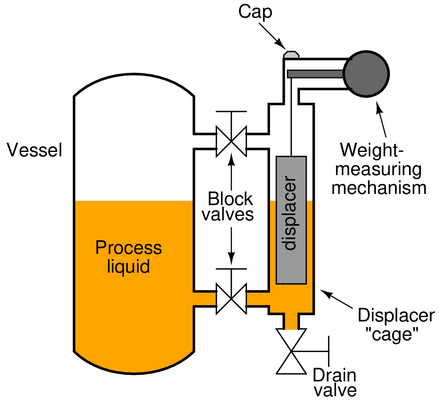Often, the displacer is housed inside its own cage’’ for easy removal from the process, as shown above, or it may be inserted directly into the process vessel like this:If the vessel is open and the liquid inside is turbulent due to mixing or other agitation, a {\it stilling well} serves the same purpose as a cage:To calibrate such an instrument, it must be isolated from the process liquid. Sometimes this means simply closing block valves and draining the cage. Other times it means removing the displacer mechanism from the vessel entirely. But once the displacer is hanging dry, there is the problem of simulating a 100

Describe a way to make the displacer think’’ it is fully submerged in process liquid when it in fact is hanging freely in the air. Explain how you would be able to {\it precisely} and {\it accurately} simulate this condition, as well as any given condition of partial displacer submersion for that matter.

Reveal answer
• #### Question 5

The following storage vessel holds water. The hydrostatic-pressure level transmitter is located 5 feet below the bottom of the vessel, and the desired level measurement range is 8 feet to 12 feet: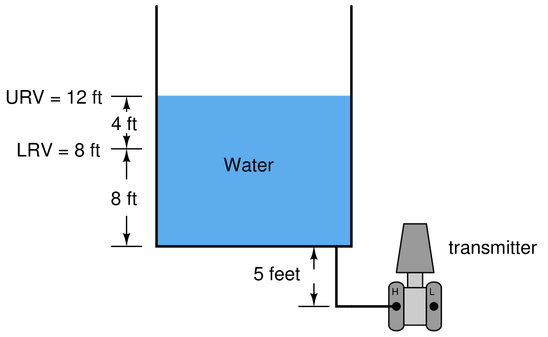Assuming a pneumatic transmitter with an output range of 3 PSI to 15 PSI, and a calibration accuracy of +/- 1

$$\begin{array} {|l|l|} \hline Process & Percent of & \Delta pressure & Output signal & Output signal & Output signal \\ \hline level (ft) & span ( \\ \hline \ & 0 & & & & \\ \hline & 10 & & & & \\ \hline & 25 & & & & \\ \hline & 50 & & & & \\ \hline & 75 & & & & \\ \hline & 90 & & & & \\ \hline & 100 & & & & \\ \hline \end{array}$$

Reveal answer
• #### Question 6

Determine the LRV and URV settings for the water seal drum lever transmitter (LT-21), assuming the LRV point is at the lower nozzle and the URV point is at the upper nozzle (the two nozzles being 3 feet 8 inches apart from each other), and that the remote seal fill fluid has a specific gravity of 0.934:Reveal answer
• #### Question 7

Calculate the hydrostatic pressure generated at the bottom of this vessel (in units of PSI) when it is completely filled with water: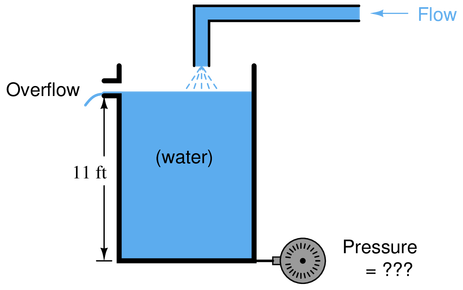Now calculate the hydrostatic pressure at the bottom of this vessel (in units of PSI) when it is completely filled with gasoline (density = 42 lb/ft$^{3}$):What do you think the pressure will be at the bottom of the vessel if it is exactly half-full of gasoline and half-full of water, with a gasoline-water {\it interface} at the 5.5 foot mark? Explain your reasoning.Reveal answer
• #### Question 8

Calculate the differential pressure sensed by the level transmitter at three different water levels in this boiler steam-drum level measurement system: 0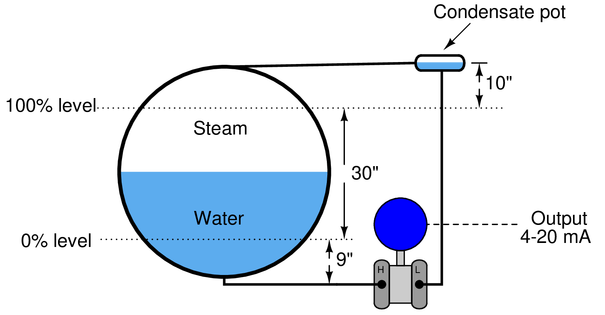Assume a density for (hot) boiler drum water of 36 lb/ft$^{3}$, a density for steam in the drum of 7 lb/ft$^{3}$, and a density for (warm) water in the wet leg’’ of 61.8 lb/ft$^{3}$. If the pressure at the low’’ (L) side of the transmitter is greater than the pressure at the high’’ (H) side, be sure to express the differential pressure quantity as a negative number.

Credit will be given for correctly calculating each of the differential pressures:

{\bullet} {\bf (6 points)} Transmitter $\Delta$P at 0
{\bullet} {\bf (6 points)} Transmitter $\Delta$P at 50
{\bullet} {\bf (6 points)} Transmitter $\Delta$P at 100

Reveal answer
• #### Question 9

A displacer-type density transmitter registers a displacer weight of 6.3 pounds with the cage completely full of sample liquid. The displacer has a dry weight of 14 pounds, a density of 120 lb/ft$^{3}$, and is cylindrical in shape. Calculate the density of the liquid in units of pounds per cubic foot.

Reveal answer
• #### Question 10

Determine a basic 5-point (0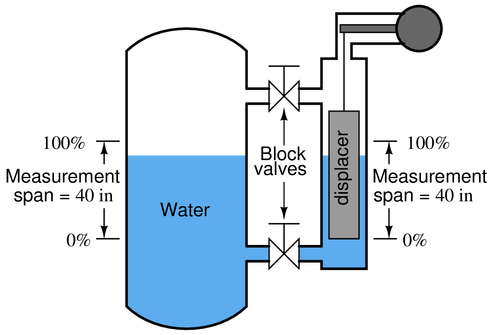The cylindrical displacer weighs 15 pounds (dry) and has a diameter of 2 inches. The process liquid is water. The 0

$$\begin{array} {|l|l|} \hline Process & Percent of & Buoyant & Output signal \\ \hline level (in) & span ( \\ \hline \ & 0 & & \\ \hline & 25 & & \\ \hline & 50 & & \\ \hline & 75 & & \\ \hline & 100 & & \\ \hline \end{array}$$

Reveal answer
• #### Question 11

Determine a basic 5-point (0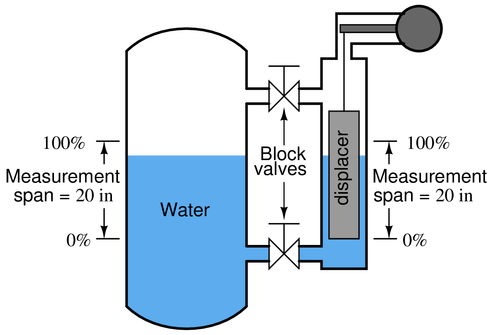The cylindrical displacer weighs 9 pounds (dry) and has a diameter of 2 inches. The process liquid is water. The 0

$$\begin{array} {|l|l|} \hline Process & Percent of & Buoyant & Output signal \\ \hline level (in) & span ( \\ \hline \ & 0 & & \\ \hline & 25 & & \\ \hline & 50 & & \\ \hline & 75 & & \\ \hline & 100 & & \\ \hline \end{array}$$

Reveal answer

### Related Content

• Share

Published under the terms and conditions of the Creative Commons Attribution License

0 Comments